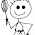## Monday, October 9, 2017

### The impracticality of the Seven Gods

1st: "The Sleeper" sounds more like the title of a Wood Allen movie than the name of a potential world builder. Sleepy's name will be changed to Luna, 'cause that'll cover it.

2nd: The impracticality: As written, none of these guys have any god-like qualities. The Seven (Dwarf) Gods,  are more of an extended family than a team of  titans. There's one responsible adult, one grumpy old man and five children. Which, now that I think about it, resembles the Greek pantheon.

3rd: Luckily, I have just uncovered some old notes for a group of five nature gods. They are:

A u t u m n,   T h e   M o t h e r   i s   t h e   s e a s o n a l   g o d d e s s   o f   h a r v e s t   a n d   w i n d .

W i n t o n,  T h e  F a t h e r  i s  t h e  s e a s o n a l  g o d  o f  w i n t e r.  H e  i s  a s s o c i a t ed  w i t h
c o l d  w e a t h e r ,  s n o w  a n d   i c e .  E x a m p l e s  o f  W i n t o n' s  a n i m a l  a n d  p l a n t
a s s o c i a t e s   a r e   w o l v e s, bears  a n d   e v e r g r e e n   t r e e s .

G a l e,   s e a s o n a l   g o d d e s s   o f   s p r i n g,  a s s o c i a t e d  w i t h  y o u t h  a n d  f e r t i l i t y.
H e r   p l a n t   a n d   a n i m a l   a s s o c i a t e s   i n c l u d e   f l o w e r i n g  t r e e s,  robins and sparrows.

W e i l d e n,   s e a s o n a l   g o d   o f   s u m m e r,  a s s o c i a t e d   w i t h   y o u n g
m a n h o o d   a n d   t h e  f r e e d o m  a n d  r e c k l e s s n e s s  o f   y o u t h .  H i s  p l a n t
a n d  a n i m a l  associates  include  b l a c k b e r r i e s   a n d   b e e s .

O r g  the unwise   o r   T h e   D e c e i v e r   i s   t r a d i t i o n a l l y   t h o u g h t   o f   a s   m a l e.
Org  i s  t h e  r e p r e s e n t a t i o n  o f   f e a r,  violence,  injustice  and  war.

4th: "Org," that's a stupid name. Regardless, now we have The Seven, The Five and the Twelve Gods. Let's hope they don't become angry gods!

1.2.